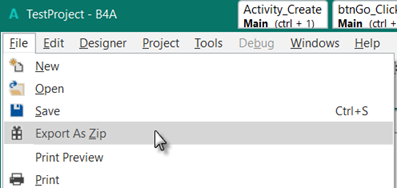# Android QuestionApp for job

#### StoopKid8978

##### Member
Hey everyone! I’ve been trying to make a simple app for my job to make things slightly more efficient. I'm new to B4A and honestly android all together. So please forgive me if this is asking too much, but I’ve been beating my head against the wall and can’t seem to figure this out. I wrote a simple ahk for the task but would like it on my phone. I want to enter a number then it subtract that number from the multiple of 24 that's above it. I’ve poured over the documentation not even sure where to start so if someone could help me I would greatly appreciate it. Please dumb it down for me, for I feel like it's the only way I will grasp it. I'll post code below...

Sub Process_Globals
'These global variables will be declared once when the application starts.
'These variables can be accessed from all modules.

End Sub

Sub Globals
'These global variables will be redeclared each time the activity is created.
'These variables can only be accessed from this module.

Private btn1 As Button
Private et1 As EditText
Private lblresult As Label

Private p1 As Int = 24
Private p2 As Int = 48
Private p3 As Int = 72
Private p4 As Int = 96
Private p5 As Int = 120
Private p6 As Int = 144
Private p7 As Int = 168
Private p8 As Int = 192
Private p9 As Int = 216
Private p10 As Int = 240

Private a2 As Int
Private a3 As Int
Private a4 As Int
Private a5 As Int
Private a6 As Int
Private a7 As Int
Private a8 As Int
Private a9 As Int
Private a10 As Int
End Sub

Sub Activity_Create(FirstTime As Boolean)

End Sub

Sub Activity_Resume

End Sub

Sub Activity_Pause (UserClosed As Boolean)

End Sub

Sub et1_EnterPressed

p1 = 24
p2 = 48
a2 = et1 - p1

If et1 < p1 Then
lblresult.Text = et1
End If
If et1 < p2
Else If et1 > p1 Then
lblresult.Text = a2
End If <---- ERROR GETS THROWN HERE
End Sub
ALSO TRIED USING
If et1 > p1 And < p2 Then
lblresult.Txt = a2
Sub btn1_Click

End Sub

#### Erel

##### B4X founder
Staff member
Longtime User
1. Please use [code]code here...[/code] tags when posting code.
2. Post the full error message from the logs. Right click to copy.

#### Brandsum

##### Well-Known Member
Sub et1_EnterPressed

p1 = 24
p2 = 48
a2 = et1 - p1
As the et1 variable is an input field, you should use et1.text to get the value of that input field

#### aeric

##### Expert
Longtime User
B4X:
``````If et1 < p1 Then
lblresult.Text = et1
End If

If et1 < p2 Then 'If et1 < p2 <-- Missing Then

Else If et1 > p1 Then
lblresult.Text = a2
End If  '<---- ERROR GETS THROWN HERE

' ALSO TRIED USING
If et1 > p1 And et1 < p2 Then ' If et1 > p1 And < p2 Then <-- Missing et1
lblresult.Txt = a2
End If

Sub btn1_Click

End Sub``````

#### klaus

##### Expert
Longtime User
There are several errors in your code.
This works
B4X:
``````Sub edtInput_EnterPressed
p1 = 24
p2 = 48
a2 = edtInput.Text - p1

If edtInput.Text < p1 Then
lblResult.Text = edtInput.Text
Else If edtInput.Text >= p1 And edtInput.Text <= p2 Then
lblResult.Text = a2
Else 'edtInput > p2
lblResult.Text = edtInput.Text
End If
End Sub``````
Did you have a look at the B4X Documentation Booklets ?
In the B4X Getting started booklet, the example project MyFirstProgram, does something similar to what you want to do.
You might also watch the B4X Video Tutorials.

Last advice: Instead of posting the code, it would be easier for us if you posted your project as a zip file.•Erel

#### StoopKid8978

##### Member
Thanks to all of you I think I finally got it.
Sorry about that. Won't happen again Erel